Question

# Solution Stoichiometry Hydrochloric acid (HCl) reacts with sodium carbonate (Na2CO3), forming sodium chloride (NaCl), water (H2O),...

Solution Stoichiometry

Hydrochloric acid (HCl) reacts with sodium carbonate (Na2CO3), forming sodium chloride (NaCl), water (H2O), and carbon dioxide (CO2). This equation is balanced as written:

2HCl(aq)+Na2CO3(aq)→2NaCl(aq)+H2O(l)+CO2(g)

a)

What volume of 2.75 M HCl in liters is needed to react completely (with nothing left over) with 0.750 L of 0.300 M Na2CO3?

b)

A 565-mL sample of unknown HCl solution reacts completely with Na2CO3 to form 10.1 g CO2. What was the concentration of the HCl solution?

How do I solve these? Thanks

2HCl(aq) + Na2CO3(aq) → 2NaCl(aq) + H2O(l) + CO2(g)

From above equation, we can say that two moles of HCl are required to neutralize Na2CO3.

We already know that, Na2CO3 is dissociated in water completely as Na+ and CO3-2 ions.

We also know that HCl is monobasic strong acid and ionizes in aqueous media in 1:1 ratio as below:

HCl(aq) + H2O(l) → H3O+(aq) + Cl-(l)

So thecomplete ionic equation is 2 H+ (aq) + 2 Cl-(l) + 2 Na+ (aq) + CO32- (aq) → H2CO3 + 2 Cl-(l) + 2 Na+ (aq)

So, here Na= and Cl- ions are spectator ions. So Net Ionic reaction is

2 H+ (aq) + CO32- (aq) → H2CO3

c = nsolute / Vsolution ⇒ nsolute = c⋅Vsolution

Here,

nCO3-2 = 0.300 mol L−1 X 0.750L= 0.225 moles CO3-2

According to the above mentioned 1:2 mole ratio, a complete neutralization would require

0.225 moles CO3-2  X2 moles H3O+ / 1 mole CO3-2 = 0.450 moles H3O+

Vsolution = nsolute / c

Therefore, VH3O+= 0.450 moles / 2.75 mol L−1 = 0.1636 L of 2.75 M HCl is required to to react completely (with nothing left over) with 0.750 L of 0.300 M Na2CO3.

Now, as discussed above we know that balance reaction is 2 HCl + Na2CO3 -----> CO2 + H2O + NaCl

Therefore,

moles of CO2  = weight / molecular weight

= 10.1 gm / 44 gm/mole = 0.22954

From balanced reaction we can say that 2 moles HCl react to form 1 mole of CO2 , therefore 0.22954 * 2 = 0.4591 moles HCl were reacted.

Now, moles= L x M

Therefore,

0.4591 moles HCl = 0.565 x M

M HCl = 00.4591 / 0.565

= 0.8126 M

So, the concentration of the HCl solution is 0.8126 M.

#### Earn Coins

Coins can be redeemed for fabulous gifts.

Similar Homework Help Questions
• ### Sodium carbonate, Na2CO3, reacts with hydrochloric acid, HCl, to produce sodium chloride, carbon dioxide and water....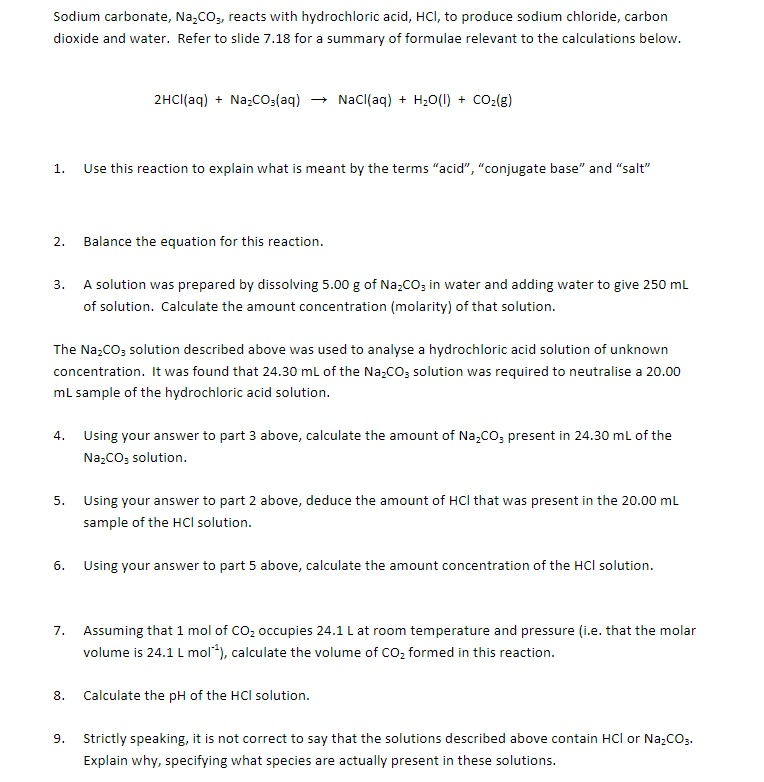Sodium carbonate, Na2CO3, reacts with hydrochloric acid, HCl, to produce sodium chloride, carbon dioxide and water. Refer to slide 7.18 for a summary of formulae relevant to the calculations below. 2HCl(aq) + Na.CO3(aq) + NaCl(aq) + H2O(l) + CO2(g) 1. Use this reaction to explain what is meant by the terms "acid", "conjugate base" and "salt" 2. Balance the equation for this reaction. 3. A solution was prepared by dissolving 5.00 g of Na2CO3 in water and adding water to...

• ### 1. What volume of 1.00 M HCl in liters is needed to react completely (with nothing...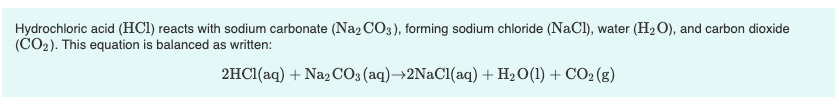1. What volume of 1.00 M HCl in liters is needed to react completely (with nothing left over) with 0.750 L of 0.200 M Na2CO3? Express your answer to three significant figures and include the appropriate units. 2. A 639-mL sample of unknown HCl solution reacts completely with Na2CO3 to form 15.1 g CO2. What was the concentration of the HCl solution? Express your answer to three significant figures and include the appropriate units. Hydrochloric acid (HCl) reacts with sodium...

• ### The stoichiometric relationship between the reactants and products of an aqueous reaction can be used to...

The stoichiometric relationship between the reactants and products of an aqueous reaction can be used to determine different kinds of information about a reaction, such as the volume of a given molarity reactant required to produce a certain amount of product. Hydrochloric acid (HCl) reacts with sodium carbonate (Na2CO3), forming sodium chloride (NaCl), water (H2O), and carbon dioxide (CO2). This equation is balanced as written: 2HCl(aq)+Na2CO3(aq)→2NaCl(aq)+H2O(l)+CO2(g) a. What volume of 2.50 M HCl in liters is needed to react completely...

• ### Sodium bicarbonate reacts with hydrochloric acid in a gas-forming reaction to produce aqueous sodium chloride, water,...

Sodium bicarbonate reacts with hydrochloric acid in a gas-forming reaction to produce aqueous sodium chloride, water, and carbon dioxide gas: NaHCO3(s) + HCl(aq) → NaCl(aq) + H2O(l) + CO2(g) Determine the mass of CO2 gas produced when 8.67 g of NaHCO3 is added to a solution that contains 5.65 g of HCl. ___g?

• ### Sodium carbonate, Na2COs, reacts with hydrochloric acid, HCl, to produce sodium chloride, carbon dioxide and water....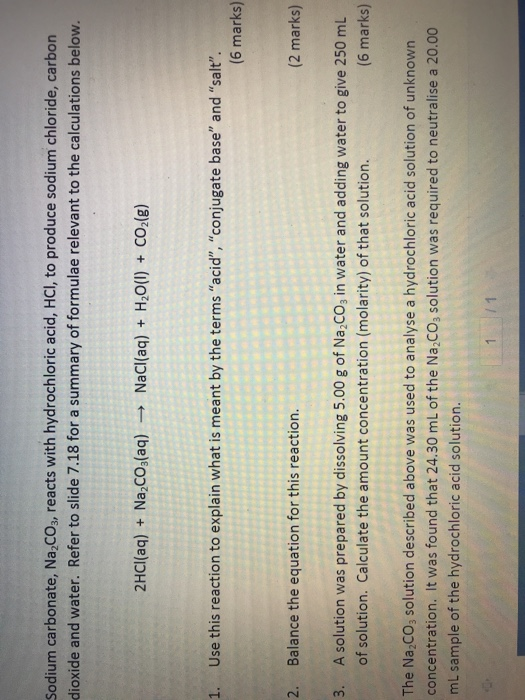Sodium carbonate, Na2COs, reacts with hydrochloric acid, HCl, to produce sodium chloride, carbon dioxide and water. Refer to slide 7.18 for a summary of formulae relevant to the calculations below. 2HCI(aq) Na,cO3(aq) NaCl(aq) H2O(I) CO2(g) 1. Use this reaction to explain what is meant by the terms "acid", "conjugate base" and "salt". (6 marks) 2 Balance the equation for this reaction. (2 marks) A solution was prepared by dissolving 5.00 g of Na,CO3 in water and adding water to give...

• ### Sodium carbonate (MM=105.988 g/mol) is a primary standard base that reacts with hydrochloric acid as follows:

Sodium carbonate (MM=105.988 g/mol) is a primary standard base that reacts with hydrochloric acid as follows: Na2CO3 + 2HCI → 2NaCl + H2O + CO2(g) If 39.09 mL of an HCl solution were required to titrate a solution containing 287.5 mg of primary standard Na2CO3, calculate the molarity of the HCl solution.

• ### 9 of 28 A Review Constants | Periodic Table 2HCl(aq) + Na2CO3(aq) +2NaCl(aq) + H2O(l) +...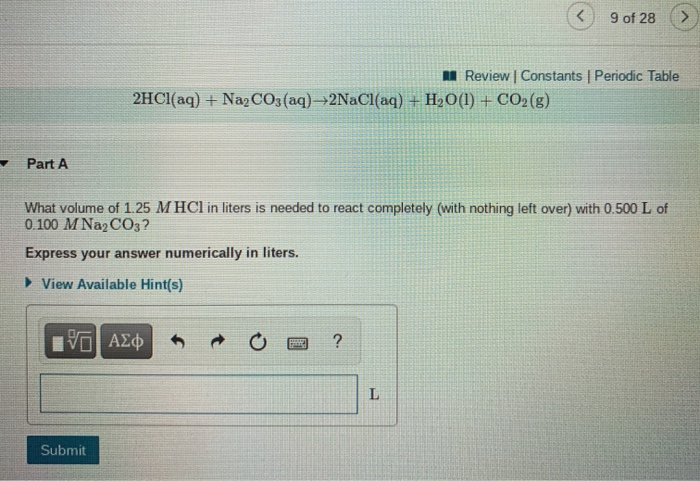9 of 28 A Review Constants | Periodic Table 2HCl(aq) + Na2CO3(aq) +2NaCl(aq) + H2O(l) + CO2(g) Part A What volume of 1.25 M HCl in liters is needed to react completely (with nothing left over) with 0.500 L of 0.100 M Na2CO3? Express your answer numerically in liters. ► View Available Hint(s) ΟΙ ΑΣΦ ? L Submit 9 of 28 Review | Constants Periodic Table Part B A 381-mL sample of unknown HCl solution reacts completely with Na2CO3 to...

• ### Calcium carbonate (CaCO3) reacts with stomach acid (HCl, hydrochloric acid) according to the following equation: CaCO3(s)...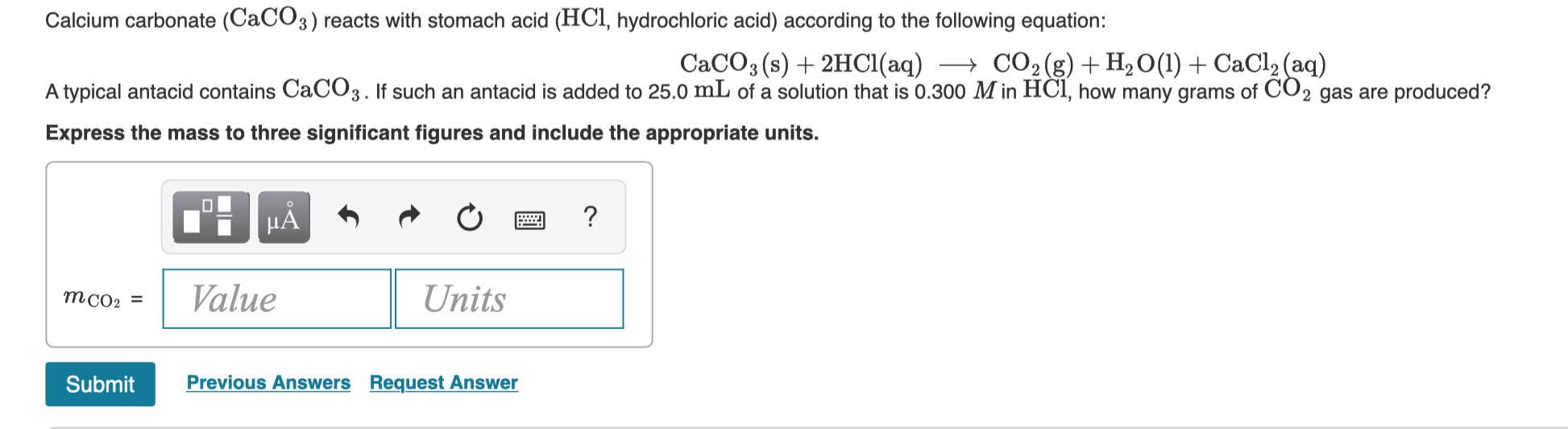Calcium carbonate (CaCO3) reacts with stomach acid (HCl, hydrochloric acid) according to the following equation: CaCO3(s) + 2HCl(aq) + CO2(g) + H2O(l) + CaCl2(aq) A typical antacid contains CaCO3. If such an antacid is added to 25.0 mL of a solution that is 0.300 M in HCl, how many grams of CO2 gas are produced? Express the mass to three significant figures and include the appropriate units. TI MÃ + + + a ? Value Units MCO: = Submit Previous...

• ### 4.00 g Na2CO3 is dissolved in H2O and titrated with HCl. 48.0 mL of a HCl...

4.00 g Na2CO3 is dissolved in H2O and titrated with HCl. 48.0 mL of a HCl solution were required to titrate the Na2CO3 solution. What is molarity of HCl solution? Na2CO3(aq) + 2HCl(aq) --> 2NaCl(aq) + H2O(l) + CO2(g)

• ### Calcium carbonate (CaCO3) reacts with stomach acid (HCl, hydrochloric acid) according to the following equation: CaCO3(s)+2HCl(aq)⟶CO2(g)+H2O(l)+CaCl2(aq)...

Calcium carbonate (CaCO3) reacts with stomach acid (HCl, hydrochloric acid) according to the following equation: CaCO3(s)+2HCl(aq)⟶CO2(g)+H2O(l)+CaCl2(aq) Tums, an antacid, contains CaCO3. If Tums is added to 10.0 mL of a solution that is 0.400 M in HCl, how many grams of CO2 gas are produced?